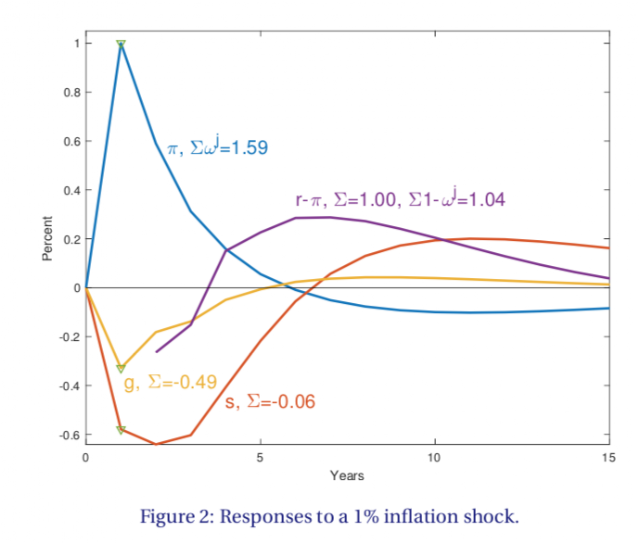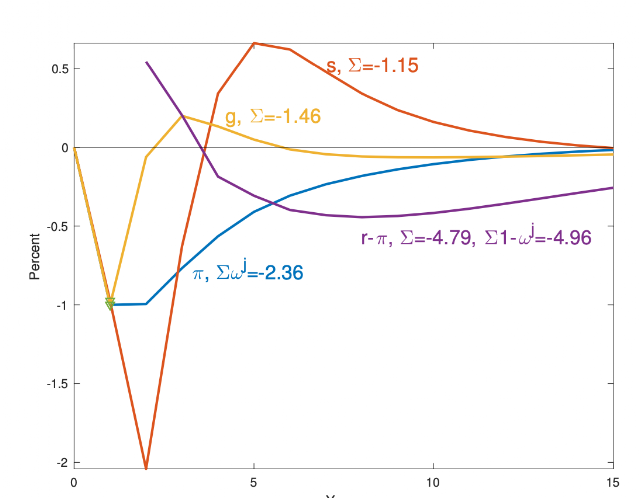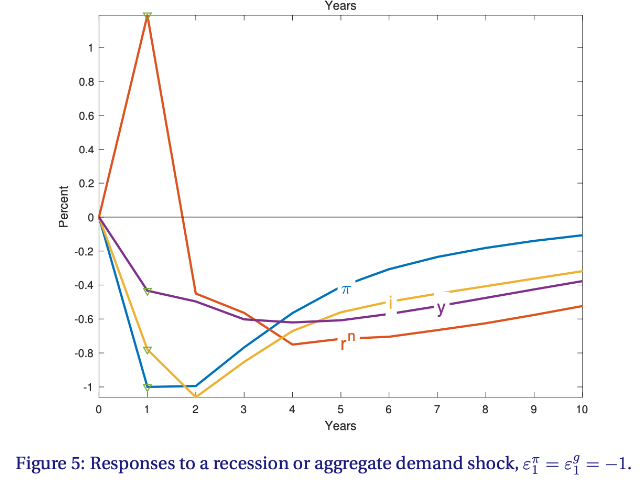# New Paper -- the fiscal roots of inflation - Barokong

I recently finished drafts of a few academic papers that blog readers might find interesting. Today, "The Fiscal Roots of Inflation."

The government debt valuation equation says that the real value of nominal debt equals the present value of surpluses. So, when there is inflation, the real value of nominal debt declines. Does that decline come about by lower future surpluses, or by a higher discount rate? You can guess the answer -- a higher discount rate.

Though to me this is interesting for how to construct fiscal theory models in which changes in the present value of government debt cause inflation, the valuation equation is every bit as much a part of  standard new-Keynesian models. So the paper does not take a stand on causality.

Here is an example of the sort of puzzle the paper addresses. Think about 2008. There was a big recession. Deficits zoomed, through bailout, stabilizers, and deliberate stimulus. Yet inflation.. declined. So how does the government debt valuation equation work? Well, maybe today's deficits are bad, but they came with news of better future surpluses. That's hard to stomach. And it isn't true in the data. Well, real interest rates declined and sharply. The discount rate for government debt declined, which raises the value of government debt, even if expected future surpluses are unchanged or declined. With a lower discount rate, government debt is more valuable. If the price level does not change, people want to buy less stuff and more government debt. That's lower aggregate demand, which pushes the price level down. Does this story bear out, quantitatively, in the data? Yes.

If you don't like discount rates and forward looking behavior, you can put the same observation in ex-post terms. When there is a big deficit, the value of debt rises. How, on average, does the debt-GDP ratio come back down on average? Well, the government could run big surpluses -- raise taxes, cut spending to pay off the debt. That turns out not to be the case. There could be a surge of economic growth. Maybe the stimuluses and infrastructure spending all pay off. That turns out not to be the case. Or, the real rate of return on government bonds could go down, so that debt grows at a lower rate. That turns out to be, on average and therefore predictably, the answer.

Identities

OK, to work. The paper starts by developing a Cambpell-Shiller type identity for government debt. This works also for arbitrary maturity structures of the debt. Corresponding to the Campbell-Shiller return linearization, $$\rho v_{t+1}=v_{t}+r_{t+1}^{n}-\pi_{t+1}-g_{t+1}-s_{t+1}.$$ The log debt to GDP ratio at the end of period $$t+1$$, $$v_{t+1}$$, is equal to its value at the end of period $$t$$, $$v_{t}$$, increased by the log nominal return on the portfolio of government bonds $$r_{t+1}^{n}$$ less inflation $$\pi_{t+1}$$, less log GDP growth $$g_{t+1}$$, and less the real primary surplus to GDP ratio $$s_{t+1}$$. Surpluses, unlike dividends, can be negative, so I don't take the log here. This surplus is scaled to have units of surplus to value, so a 1% change in "surplus" changes the log value of debt by 1%. I use this equation to measure the surplus.

Iterating forward, and imposing the transversality condition, we have a Campbell-Shiller style present value identity, $$v_{t}=\sum_{j=1}^{\infty}\rho^{j-1}s_{t+j}+\sum_{j=1}^{\infty}\rho^{j-1}g_{t+j} -\sum_{j=1}^{\infty}\rho^{j-1}\left( r_{t+j}^{n}-\pi _{t+j}\right).$$ Take innovations $$\Delta E_{t+1} \equiv E_{t+1}-E_t$$ and we have $$\Delta E_{t+1}\pi_{t+1}-\Delta E_{t+1} r_{t+1}^{n}= -\sum_{j=0}^{\infty} \rho^{j} \Delta E_{t+1}s_{t+1+j} -\sum_{j=0}^{\infty} \rho^{j} \Delta E_{t+1} g_{t+1+j}+\sum_{j=1}^{\infty} \rho^{j} \Delta E_{t+1}\left( r_{t+1+j}^{n}-\pi_{t+1+j}\right)$$ Unexpected inflation devalues bonds. So it must come with a decline in surpluses, a rise in the discount rate, or a decline in bond prices. Notice the value of debt disappeared, which is handy.

The bond return comes from future expected returns or inflation, so it's nice to get rid of that too. With a geometric maturity structure in which the face value of bonds of $$j$$ maturity is $$\omega^j$$, a high bond return today must come from lower bond returns in the future. $$\Delta E_{t+1}r_{t+1}^{n} = -\sum_{j=1}^{\infty}\omega^{j}\Delta E_{t+1} r_{t+1+j}^{n} =-\sum_{j=1}^{\infty}\omega^{j}\Delta E_{t+1}\left[ (r_{t+1+j}^{n}-\pi_{t+1+j})+\pi_{t+1+j}\right]$$ Substitute and we have the last and best identity $$\sum_{j=0}^{\infty}\omega^{j} \Delta E_{t+1}\pi_{t+1+j} = -\sum_{j=0}^{\infty} \rho^{j} \Delta E_{t+1}s_{t+1+j} -\sum_{j=0}^{\infty} \rho^{j} \Delta E_{t+1}g_{t+1+j} +\sum_{j=1}^{\infty} (\rho^{j} -\omega^{j})\Delta E_{t+1}\left( r_{t+1+j}^{n}-\pi_{t+1+j}\right) .$$ With long-term debt a weighted sum of current and future inflation corresponds to changes in expected surpluses and discount rates. A fiscal shock can result in future inflation, thereby falling on today's long term bonds. Equivalently, a surprise deficit today $$s_{t+1}$$ must be met by future surpluses, by lower returns, or by devaluing outstanding bonds, so that the debt/GDP ratio is reestablished.

Results

I ran a VAR and computed the responses to various shocks.Here is the response to an inflation shock - -an unexpected movement $$\Delta E_1 \pi_1$$. All other variables may move at the same time as the inflation shock.

Inflation is persistent, so a 1% inflation shock is about a 1.5% cumulative inflation shock, weighted

by the maturity of outstanding debt.

So, where is the 1.5% decline in present value of surpluses? Which terms of the identity matter?

Inflation does come with persistent deficits here. The sample is 1947-2018, so a lot of the inflation shocks come in the 1970s. You might raise three cheers for the fiscal theory, but not so fast. The deficits turn around and become surpluses. The sum of all surpluses term in the identity is a trivial -0.06, effectively zero. These deficits are essentially all paid back by subsequent surpluses.

Growth declines by half a percentage point cumulatively, accounting for 2/3 of the inflation. And the discount rate rises persistently. Two thirds of the devaluation of debt that inflation represents comes from higher real expected returns on government bonds, which in turn means higher interest rates that don't match inflation. (More graphs in the paper.)

Growth here is negatively correlated with inflation, which is true of the overall sample, but not of the story I started out with. What happens in a normal recession, that features lower inflation and lower output? Let's call it an aggregate demand shock. To measure such an event, I simply defined a shock that moves both output and inflation down by 1%. Here are the responses to this "recession shock."Inflation and output go down now, by 1%, and by construction. That's how I defined the shock. This is a recession with low growth, low inflation, and deficits. Not shown, interest rates all decline too.

So where does the low inflation come from in the above decomposition. Do today's deficits signal future surpluses? Yes, a bit. But not enough -- the cumulative sum of surpluses is -1.15% On its own, deficits should cause 1% inflation, the fiscal theory puzzle that started me out in this whole business. Growth quickly recovers, but is not positive for a sustained period. Like 2008, we see a basically downward shift in the level of GDP. That contributes another 1% inflationary force. The discount rate falls however,  so strongly as to raise the real value debt by almost 5 percentage points! That overcomes the inflationary forces and accounts for the deflation.

Here is a plot of the interest rates in response to the same shock. i is the three month rate, y is the 10 year rate, and rn is the return on the government bond portfolio. Yes, interest rates at all maturities jump down in this recession. Sharply lower rates mean a one-period windfall for the owners of long term bonds, then expected bond returns fall too.The point

Discount rates matter. If you want to understand the fiscal foundations of inflation, you have to understand the government debt valuation equation. Inflation and deflation over the cycle is not driven by changing expected surpluses. If you want to view it "passively," inflation and deflation over the cycle does not result in passive policy accommodation through taxes, as most footnotes presume. The fiscal roots (or consequences) of inflation over the cycle are the strong variation in discount rates -- expected returns.

The fiscal process

Notice that the response of primary surpluses in all these graphs is s-shaped. Primary surpluses do not follow an AR(1) type process. In response to today's deficits, there is eventually a shift to a long string of surpluses that partially repay much though not all of that debt. This seems completely normal, except that so many models specify AR(1) style processes for fiscal surpluses. Surely that is a huge mistake. Stay tuned. The next paper shows how to put an s-shaped surplus process in a model and why it is so important to do so.

Comments on the paper are most welcome.